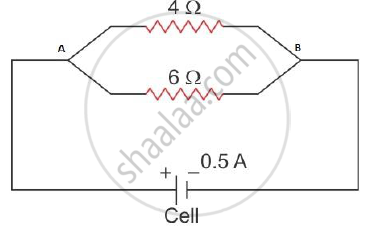Share

# Two Resistors of 4Ω and 6 Ω Are Connected in Parallel to a Cell to Draw 0.5 a Current From the Cell. - ICSE Class 10 - Physics

ConceptResistance of a System of Resistors - Resistors in Series

#### Question

Two resistors of 4Ω and 6 Ω are connected in parallel to a cell to draw 0.5 A current from the cell.
(i) Draw a labelled circuit diagram showing the above arrangement.
(ii) Calculate the current in each resistor. What is an Ohmic resistor?

#### Solution

i.

The parallel combination of two resistors 4 Ω and 6 Ω that draws a current of 0.5 A from the cell is as shown below.ii.

In parallel combination of resistors potential difference across each resistor is same as the potential difference across the terminals of the battery.
Here current drawn from cell, I = 0.5 A

The effective resistance 1/R=1/R_1+1/R_2

Here R1=4Ω and R2=6Ω

therefore 1/R=1/4+1/6

therefore 1/R=5/12

therefore R=12/5

therefore  R=2.4 Omega

Is there an error in this question or solution?

#### APPEARS IN

Solution Two Resistors of 4Ω and 6 Ω Are Connected in Parallel to a Cell to Draw 0.5 a Current From the Cell. Concept: Resistance of a System of Resistors - Resistors in Series.
S